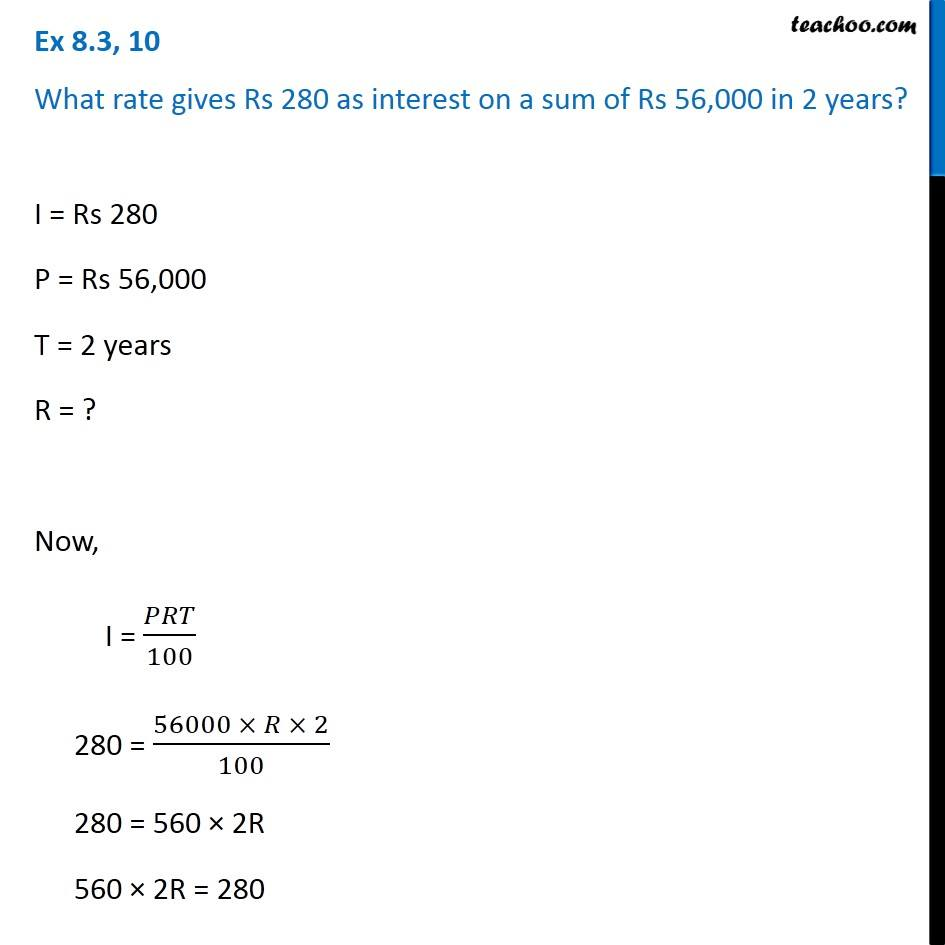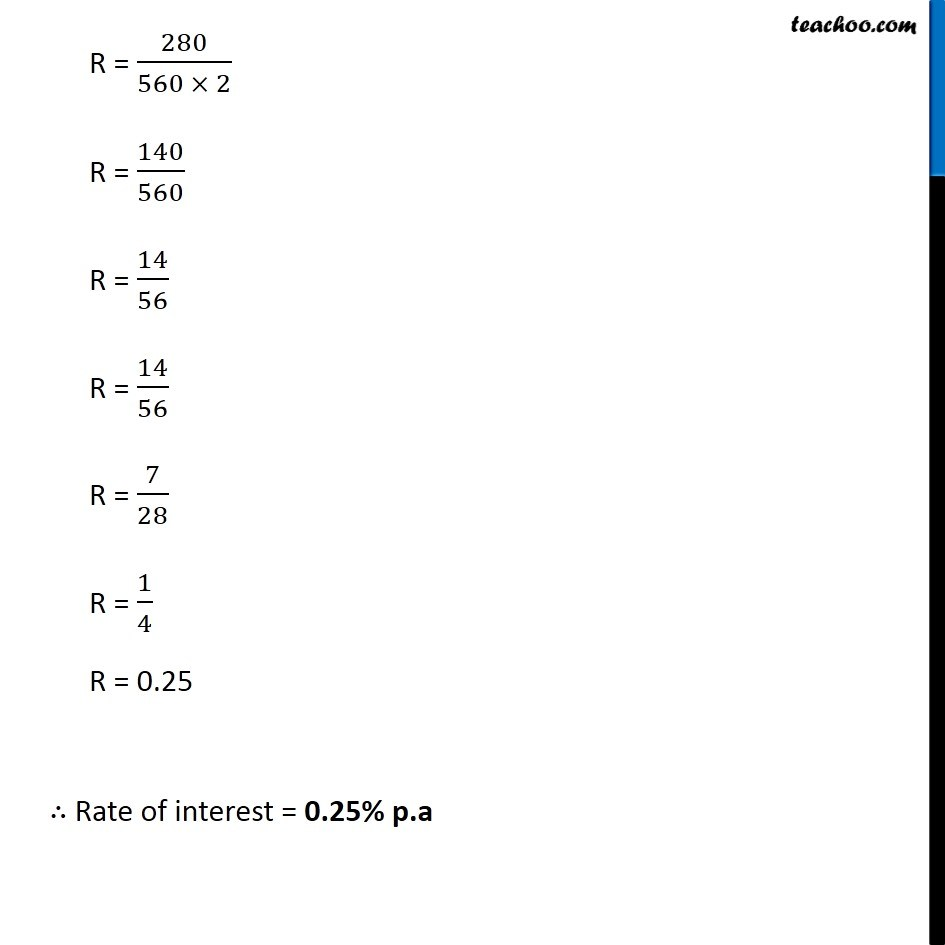Simple interest

Chapter 8 Class 7 Comparing Quantities
Concept wiseIntroducing your new favourite teacher - Teachoo Black, at only ₹83 per month

### Transcript

Ex 8.3, 10 What rate gives Rs 280 as interest on a sum of Rs 56,000 in 2 years? I = Rs 280 P = Rs 56,000 T = 2 years R = ? Now, I = 𝑃𝑅𝑇/100 280 = (56000 × 𝑅 × 2)/100 280 = 560 × 2R 560 × 2R = 280 R = 280/(560 × 2) R = 140/560 R = 14/56 R = 14/56 R = 7/28 R = 1/4 R = 0.25 ∴ Rate of interest = 0.25% p.a